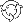The Theory \ Details \ portfolio optimization \ mean-variance criterion

# Portfolio Optimization

## Utility functions approach vs. mean-variance approach

Assume that the analytical model holds in the market with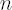assets, excess Mu vector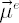and the covariance matrix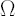. By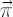we denote the vector of constant portfolio weights.

Mean-Variance Approach can be formulated in several equivalent ways.

1. Minimization of portfolio volatility subject to the lower constraint on portfolio excess Mu (over all admissible portfolios):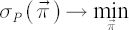subject to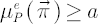2. Maximization of portfolio Excess Mu subject to the upper constraint on portfolio volatility (over all admissible portfolios):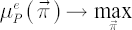subject to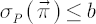, where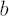is a strictly positive constant.
3. Maximization of the following expression (over all admissible portfolios):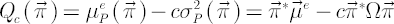, where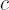is a strictly positive constant.

Utility Function Approach consists in maximizing the expected value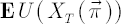of utility functionfrom portfolio terminal wealth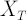over all admissible portfolios.

Key Result
Under the assumptions of the analytical model the maximization of expected CRRA utility with the relative risk aversion coefficient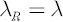from terminal wealth and the maximization of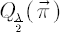(both maximizations are taken over all admissible portfolio vectors) result in the same optimal portfolio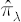.

Important!
The above statement allows all the results obtained in single-period framework to be easily extended to multi-period one with CRRA Utility Functions.

Note.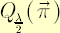is also known as the Risk-Adjusted Expected Excess Rate of Return, corresponding to the
relative risk aversion coefficient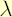.